# Odd Even Functions

The graph of equation has symmetry along the x-axis or y-axis. In this article, the symmetry of a graph of function is defined in terms of even and odd function.

## Even Function

Let’s understand the even functions first. The function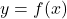is even function, if for every x in domain of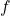.

f(-x) = f(x)

For example,

Letbe a function where

f(x) = x^2

The function will square any input value for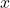and output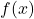. Let us put some values for, see the following table of values.

The table shows that any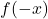values is equal to.

The graph of even function is symmetric along the y-axis.

## Odd Functions

The odd function is different from even function in terms of symmetry of graph of the function. The functionis odd function if for everyin the domain of.

f(-x) = -f(x)

Consider the following table of points for the function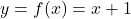.

The graph of odd function shows that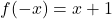is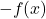.